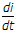# Electronics and Communication Engineering - Power Electronics

### Exercise :: Power Electronics - Section 2

31.

In a 3 phase full wave regulator feeding resistance load connected in star, the possible range of firing angle a is

 A. 0 ≤ a ≤ 180° B. 0 ≤ a ≤ 150° C. 0 ≤ a ≤ 120° D. 0 ≤ a ≤ 90°

Explanation:

Since output voltage is zero if a ≥ 150°, the possible range of firing angles is 0 ≤ a ≤ 150°.

32.

Assertion (A): A dual converter may be circulating current type or non circulating current type.

Reason (R): In circulating current dual converter only one converter can be operated at one time.

 A. Both A and R are correct and R is correct explanation of A B. Both A and R correct but R is not correct explanation of A C. A is correct but R is wrong D. A is wrong but R is correct

Explanation:

In circulating current dual converter both converters operate together.

33.

Current chopping leads to

 A. over voltage B. over current C. both over voltage and over current D. either over voltage or over current

Explanation:

e = L. In current choppingis high. Hence e is high.

34.

A 3 phase bridge inverter is fed by 400 V battery. The load is star connected and has a resistance of 10 Ω per phase. The peak value of load current is

 A. 40 A B. 20 A C. 10 A D. 5 A

Explanation:

Two resistances are in series at any time.

35.

In an SCR, the anode current is controlled by

 A. gate current only B. external circuit only C. both gate current and external circuit D. none of the above